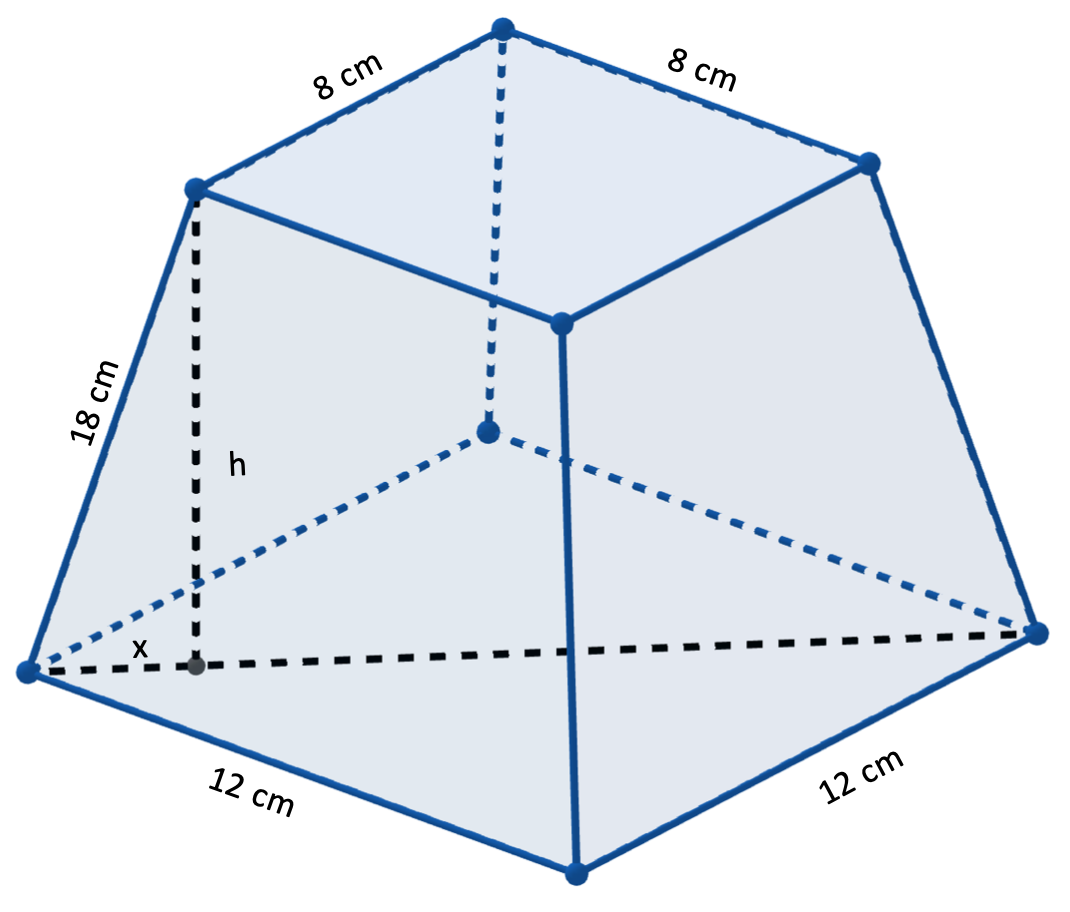# Solid mensuration

3 posts / 0 new
MONGOL○482
Solid mensuration

Good day sir!
"A frustum of a regular pyramid has a lower base of 12cm by 12cm and an upper base of 8cm by 8cm. If the lateral edge is 18cm, compute the volume of the regular pyramid."

The answer is V=1801.71cm3 which is actually the volume of the frustum. Is he correct sir? Because i was thinking i should get the whole volume of the square based regular pyramid.

Jhun Vert$2x = \sqrt{12^2 + 12^2} - \sqrt{8^2 + 8^2}$

$x = 2\sqrt{2} ~ \text{cm}$$h = \sqrt{18^2 - x^2}$

$h = 2\sqrt{79} ~ \text{cm}$

$V = \dfrac{h}{3}\left( A_1 + A_2 + \sqrt{A_1 A_2} \right)$

$V = \dfrac{2\sqrt{79}}{3}\left( 12^2 + 8^2 + \sqrt{12^2(8^2)} \right)$

$V = 1801.34 ~ \text{cm}^3$

MONGOL○482

Of course! Now i know why. Thank you sir for the hint!

Subscribe to MATHalino on